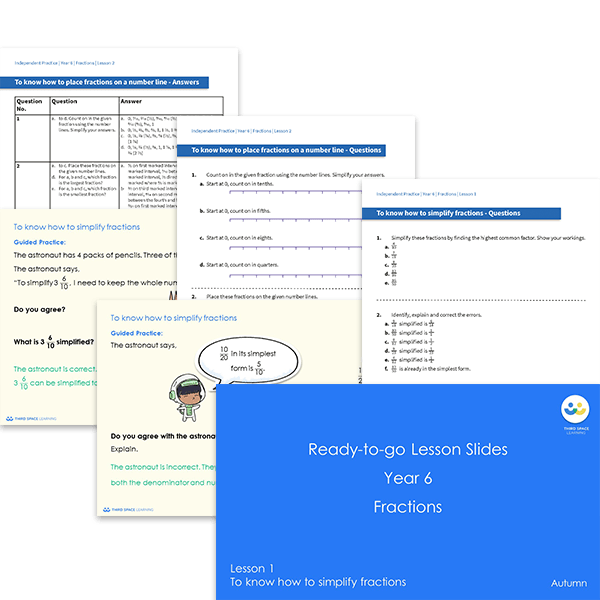# Ready-to-go lessons Year 6 Fractions (Autumn Block 3) Slides and Worksheets

Fractions ready-to-go lessons for Year 6 includes 16 PowerPoints with matching worksheets ready for you to use with a class. These resources follow a mastery approach to mathematics teaching, similar to schemes such as White Rose Maths and Mathematics Mastery. The PowerPoints are fully editable with detailed notes for every teaching slide. Each associated worksheet is divided into three parts with a range of activities to complete across the block.

This Year 6 Fractions pack contains 16 fully editable PowerPoints and 16 worksheets.

The slides and worksheets cover:

• Simplifying fractions
• Placing fractions on a number line
• Comparing and ordering fractions by their denominator or their numerator
• Adding and subtracting fractions including mixed number and improper fractions
• Solving problems involving adding and subtracting fractions
• Multiplying fractions by integers and fractions by fractions
• Dividing fractions by integers
• Using the four operations when calculating with fractions
• Finding a fraction of an amount and finding a whole from known values

Year 6

Fractions